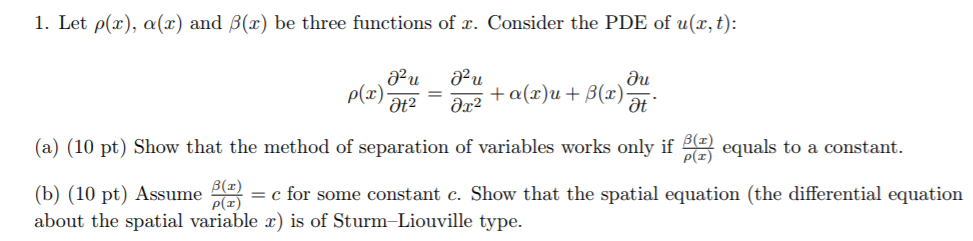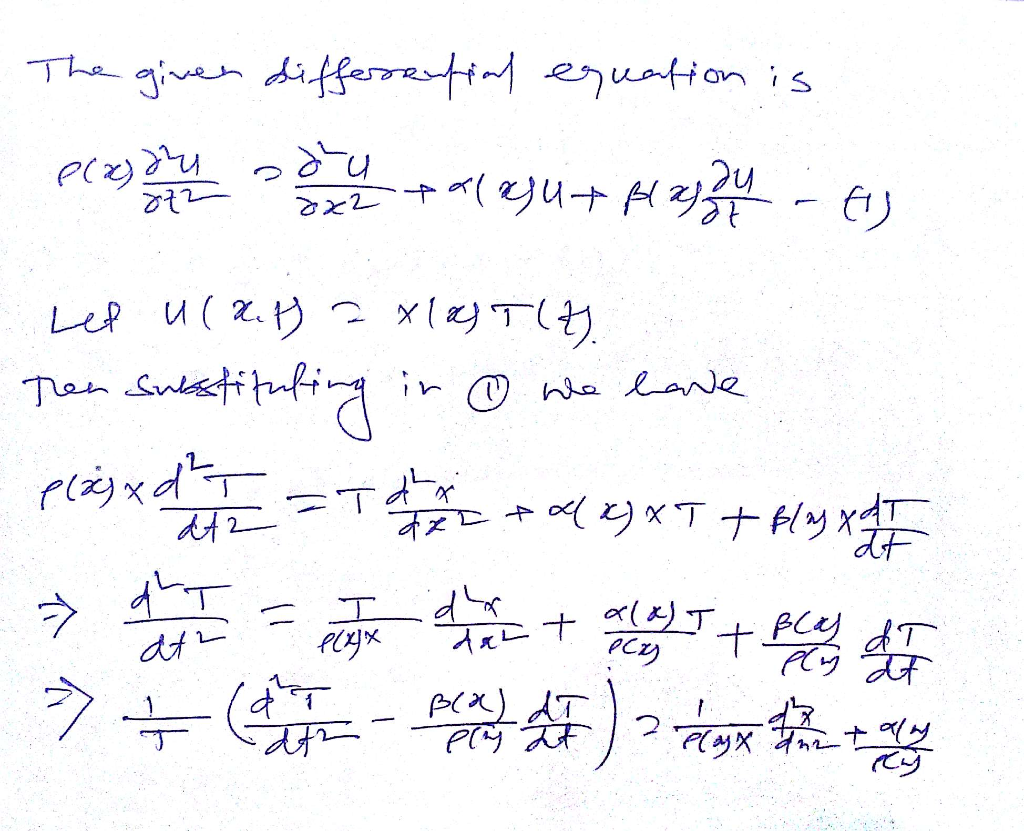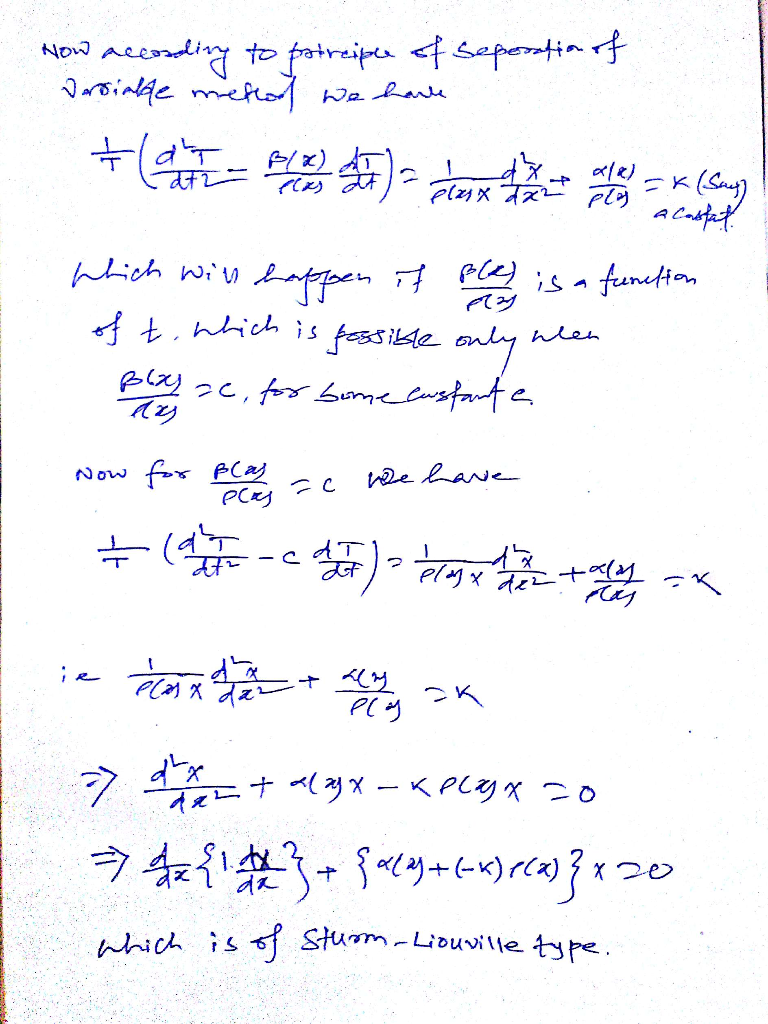Homework Help Question & Answers

# 1. Let p(x), a(x) and B(x) be three functions of r. Consider the PDE of u(r, t): PEx) at (a) (10 ...1. Let p(x), a(x) and B(x) be three functions of r. Consider the PDE of u(r, t): PEx) at (a) (10 pt) Show that the method of separation of variables works only if ρ(r) equals to a constant. (b) (10 pt) Assumefor some constant c. Show that the spatial equation (the differential equation about the spatial variable r) is of Sturm-Liouville type. B(z)

#### Homework Answers

ReportAnswer #1Add Homework Help Answer
##### Add Answer of: 1. Let p(x), a(x) and B(x) be three functions of r. Consider the PDE of u(r, t): PEx) at (a) (10 ...
Your Answer: Your Name: What's your source?
Not the answer you're looking for? Ask your own homework help question. Our experts will answer your question WITHIN MINUTES for Free.
More Homework Help Questions Additional questions in this topic.

• #### -9 2. Let Vi-8.V2,andvs-2, let B -(V,V2,Vs), and let W be the subspace spanned , let B -(Vi,V2,V3...

Need Online Homework Help?

Get FREE EXPERT Answers
WITHIN MINUTES
Related Questions